# Static Electricity Dr Walker Electrostatics Electro Electricity static

• Slides: 82Static Electricity Dr. WalkerElectrostatics • Electro – – Electricity • -static – At rest – Electric charges – Forces between charges – Behavior of electric charges in (and through) materialsAtomic Structure • Protons and neutrons stay within the nucleus. It takes a VERY large amount of energy to change that. • Electrons are often removed from and added to an atom by normal everyday occurrences due to the comparatively weak attractive force to the nucleus (even with the opposite charge)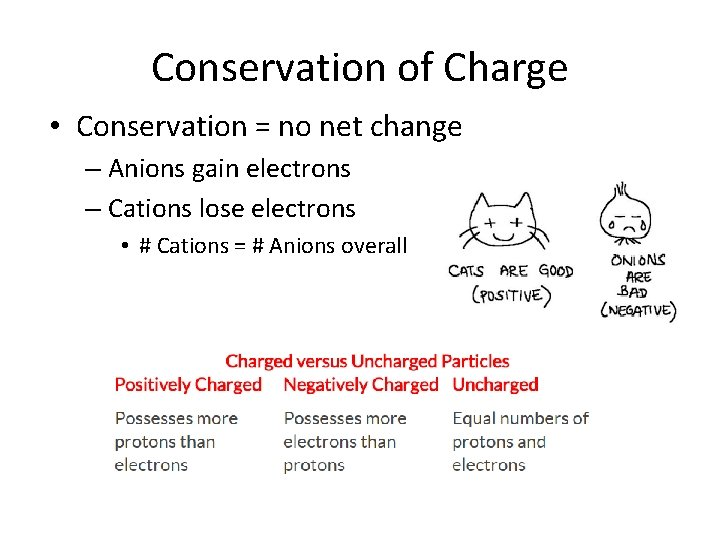Conservation of Charge • Conservation = no net change – Anions gain electrons – Cations lose electrons • # Cations = # Anions overall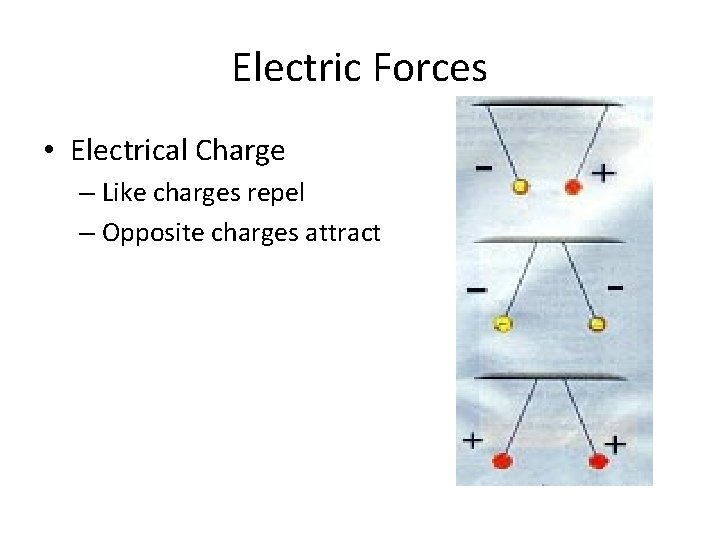Electric Forces • Electrical Charge – Like charges repel – Opposite charges attractElectrical Forces • Forces of electrical attraction and repulsion are MUCH stronger than gravity! • G (gravitational constant) = 6. 67 x 10 -11Electrical Forces • Electrical charges have a force associated with them because they have a mass – It’s just REALLY small – Electron mass is roughly 10 -31 kg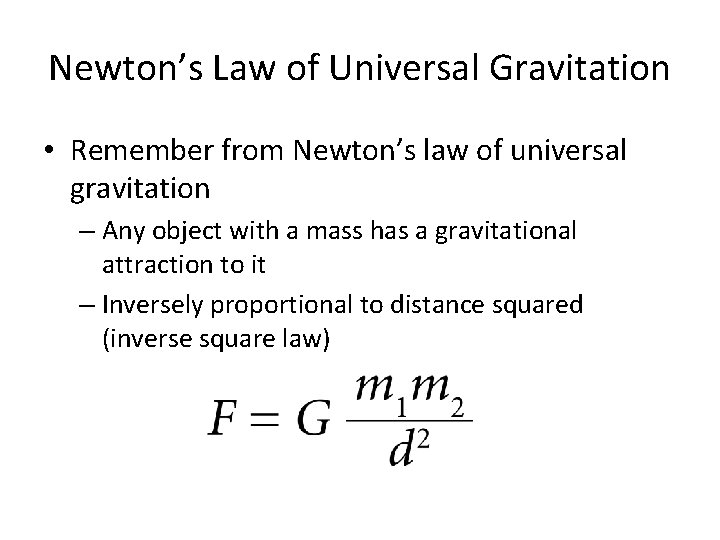Newton’s Law of Universal Gravitation • Remember from Newton’s law of universal gravitation – Any object with a mass has a gravitational attraction to it – Inversely proportional to distance squared (inverse square law)Coulomb’s Law • Electrical equivalent to Newton’s law of universal gravitation Newton – macroscopic masses Coulomb – electrical charges F = force k = constant (9. 0 × 109 N·m 2/C 2 ) q 1 and q 2 = quantities of charge d = distance (in meters)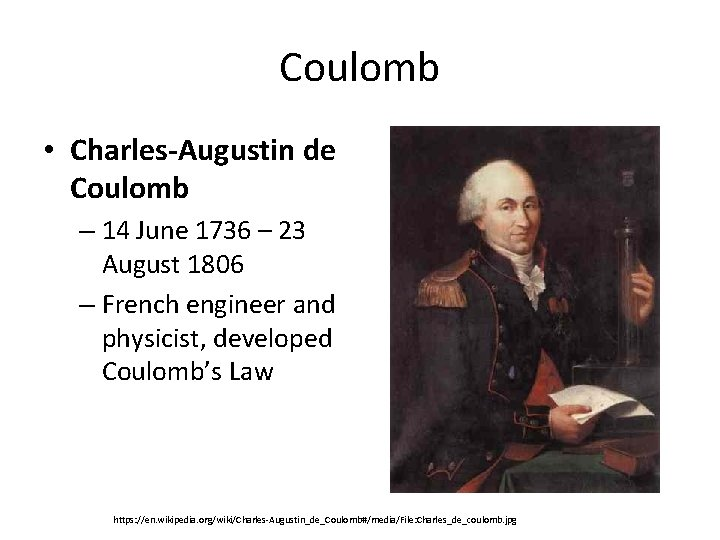Coulomb • Charles-Augustin de Coulomb – 14 June 1736 – 23 August 1806 – French engineer and physicist, developed Coulomb’s Law https: //en. wikipedia. org/wiki/Charles-Augustin_de_Coulomb#/media/File: Charles_de_coulomb. jpg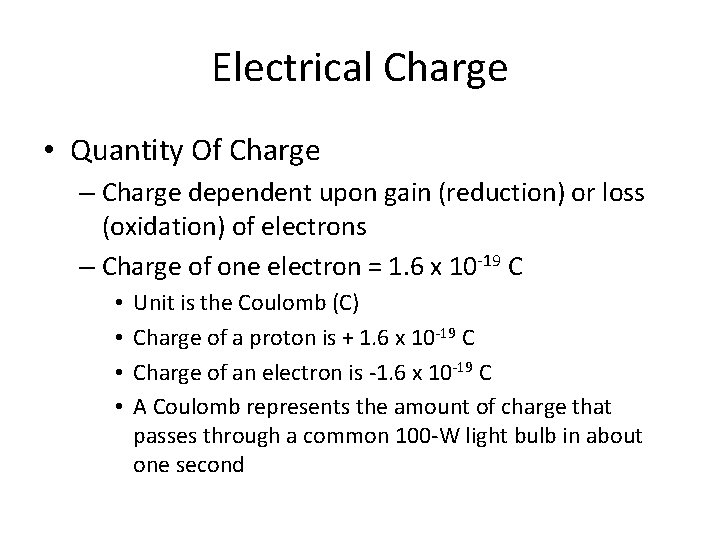Electrical Charge • Quantity Of Charge – Charge dependent upon gain (reduction) or loss (oxidation) of electrons – Charge of one electron = 1. 6 x 10 -19 C • • Unit is the Coulomb (C) Charge of a proton is + 1. 6 x 10 -19 C Charge of an electron is -1. 6 x 10 -19 C A Coulomb represents the amount of charge that passes through a common 100 -W light bulb in about one secondCoulomb’s Law Example 1 • Suppose that two point charges, each with a charge of +1. 00 Coulomb are separated by a distance of 1. 00 meter. Determine the magnitude of the electrical force of repulsion between them.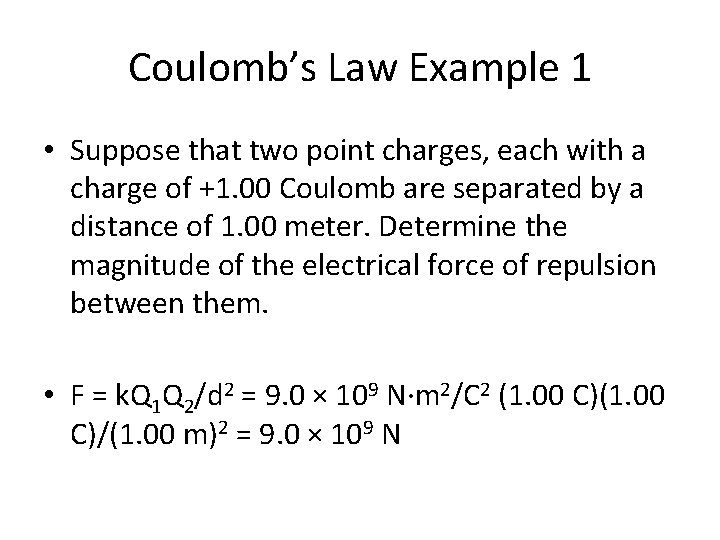Coulomb’s Law Example 1 • Suppose that two point charges, each with a charge of +1. 00 Coulomb are separated by a distance of 1. 00 meter. Determine the magnitude of the electrical force of repulsion between them. • F = k. Q 1 Q 2/d 2 = 9. 0 × 109 N·m 2/C 2 (1. 00 C)/(1. 00 m)2 = 9. 0 × 109 N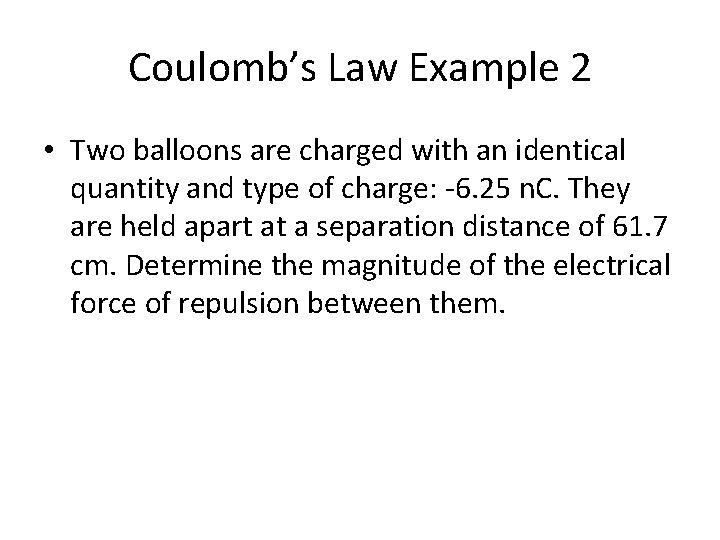Coulomb’s Law Example 2 • Two balloons are charged with an identical quantity and type of charge: -6. 25 n. C. They are held apart at a separation distance of 61. 7 cm. Determine the magnitude of the electrical force of repulsion between them.Coulomb’s Law Example 2 • Two balloons are charged with an identical quantity and type of charge: -6. 25 n. C. They are held apart at a separation distance of 61. 7 cm. Determine the magnitude of the electrical force of repulsion between them. • F = k. Q 1 Q 2/d 2 = 9. 0 × 109 N·m 2/C 2 (6. 25 x 10 -9 C)/(0. 617 m)2 = 9. 23 x 10 -7 N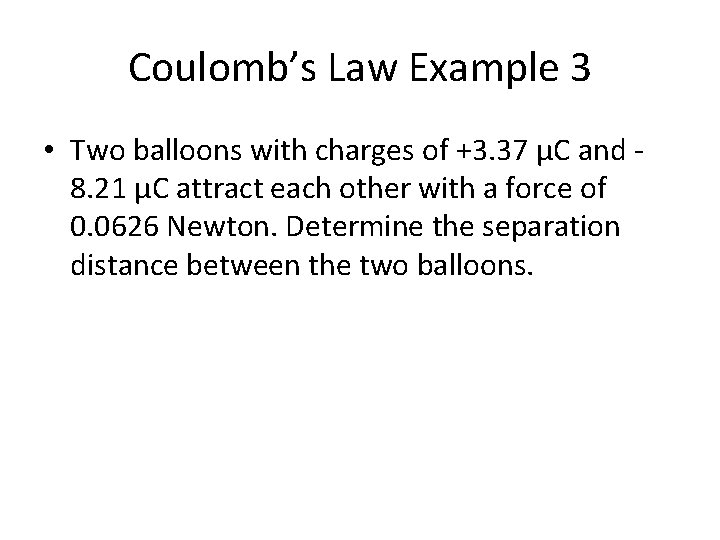Coulomb’s Law Example 3 • Two balloons with charges of +3. 37 µC and 8. 21 µC attract each other with a force of 0. 0626 Newton. Determine the separation distance between the two balloons.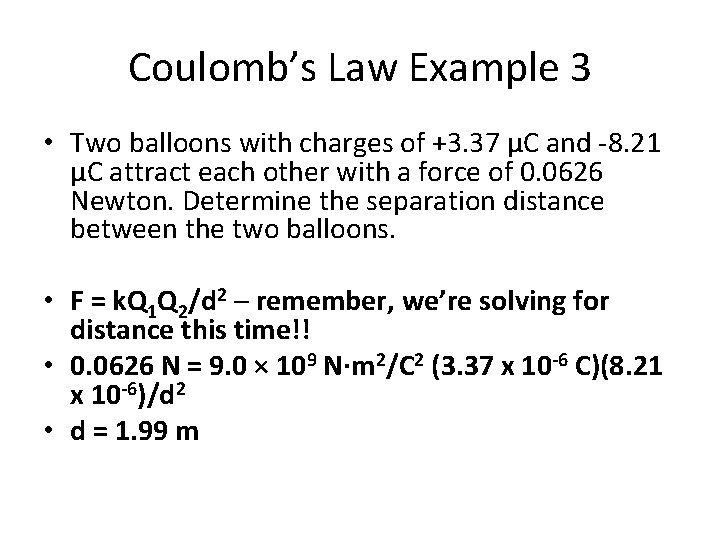Coulomb’s Law Example 3 • Two balloons with charges of +3. 37 µC and -8. 21 µC attract each other with a force of 0. 0626 Newton. Determine the separation distance between the two balloons. • F = k. Q 1 Q 2/d 2 – remember, we’re solving for distance this time!! • 0. 0626 N = 9. 0 × 109 N·m 2/C 2 (3. 37 x 10 -6 C)(8. 21 x 10 -6)/d 2 • d = 1. 99 m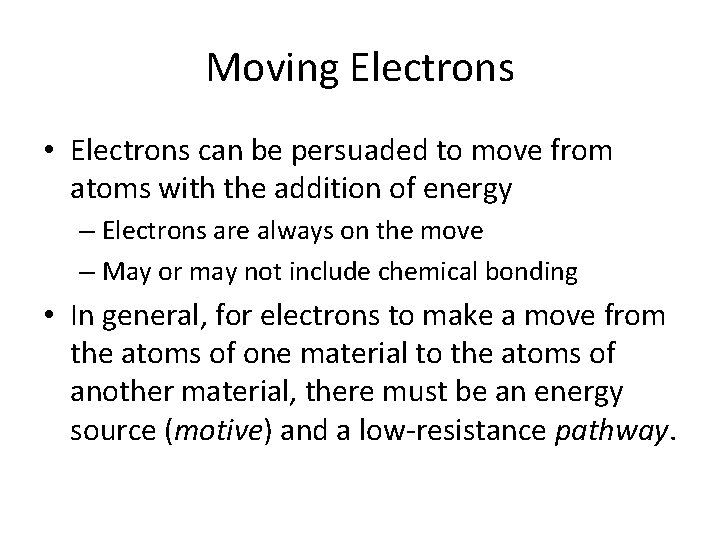Moving Electrons • Electrons can be persuaded to move from atoms with the addition of energy – Electrons are always on the move – May or may not include chemical bonding • In general, for electrons to make a move from the atoms of one material to the atoms of another material, there must be an energy source (motive) and a low-resistance pathway.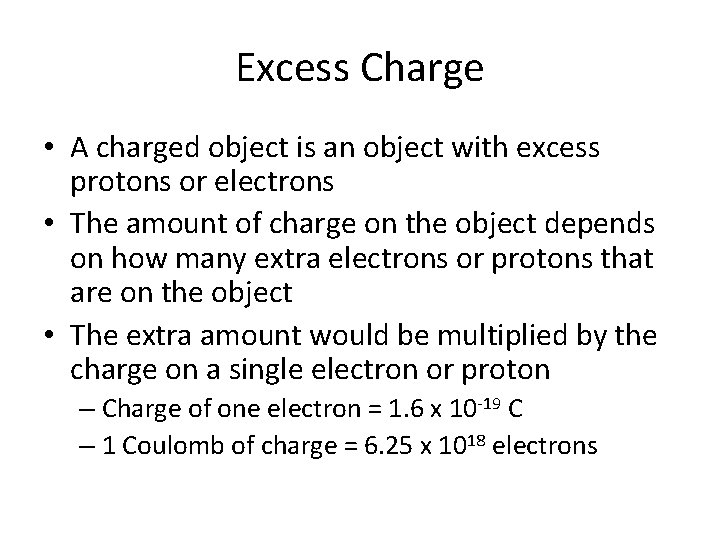Excess Charge • A charged object is an object with excess protons or electrons • The amount of charge on the object depends on how many extra electrons or protons that are on the object • The extra amount would be multiplied by the charge on a single electron or proton – Charge of one electron = 1. 6 x 10 -19 C – 1 Coulomb of charge = 6. 25 x 1018 electronsCounting Particles • The amount of charge carried by a lightning bolt is estimated at 10 Coulombs. What quantity of excess electrons is carried by the lightning bolt?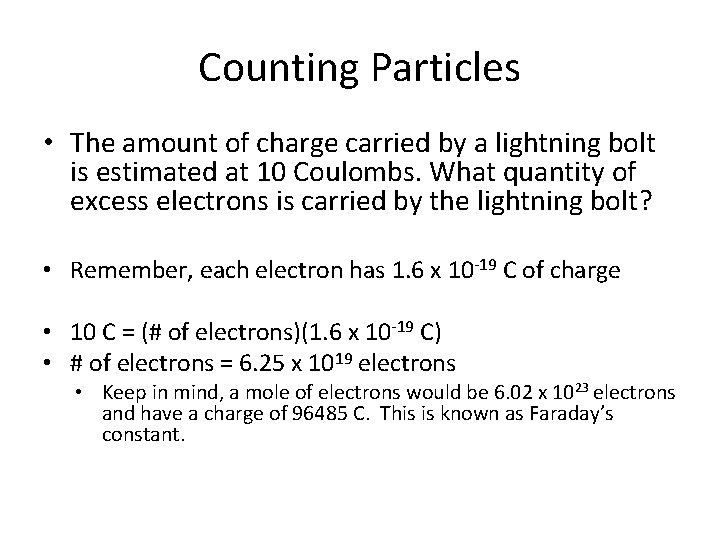Counting Particles • The amount of charge carried by a lightning bolt is estimated at 10 Coulombs. What quantity of excess electrons is carried by the lightning bolt? • Remember, each electron has 1. 6 x 10 -19 C of charge • 10 C = (# of electrons)(1. 6 x 10 -19 C) • # of electrons = 6. 25 x 1019 electrons • Keep in mind, a mole of electrons would be 6. 02 x 1023 electrons and have a charge of 96485 C. This is known as Faraday’s constant.Electron Affinity • Refers to the affinity or “love” of electrons for a certain material – Rub a balloon on animal fur or your hair – Electrons have a higher affinity for the rubber than your hair or the fur, so the rubber on the balloon takes a negative charge • On a dry day, the balloon may stick to your head • The transfer of electrons results in a difference of charge (just like in chemical bonding) and an electrostatic attraction resultsAttraction and Repulsion • Remember, like charges repel and opposite charges attract – Electrons move from hair to the balloons – Both balloons have extra electrons and will repel each other http: //www. physicsclassroom. com/class/estatics/Lesson-2/Charging-by-Friction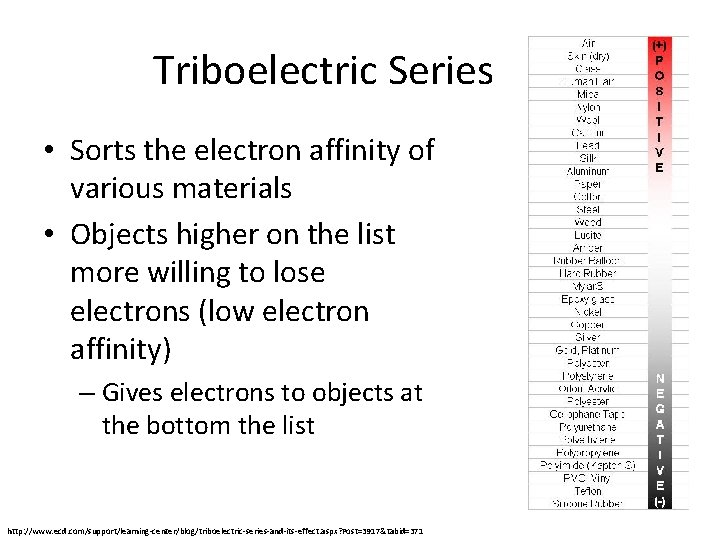Triboelectric Series • Sorts the electron affinity of various materials • Objects higher on the list more willing to lose electrons (low electron affinity) – Gives electrons to objects at the bottom the list http: //www. ecd. com/support/learning-center/blog/triboelectric-series-and-its-effect. aspx? Post=3917&tabid=371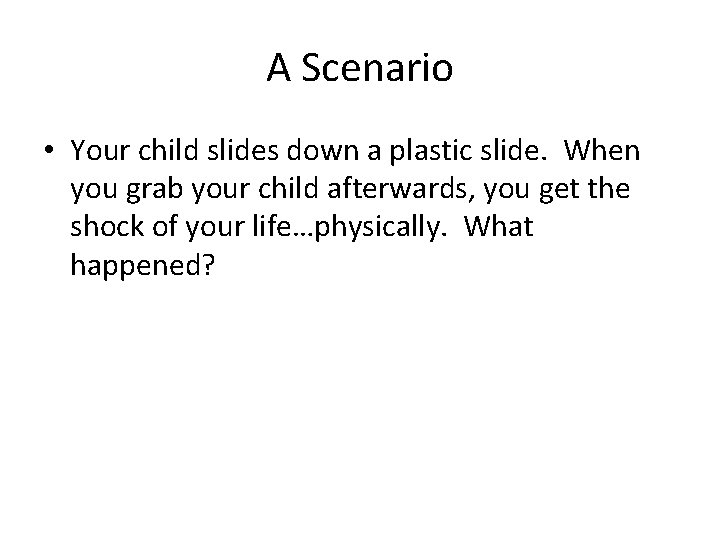A Scenario • Your child slides down a plastic slide. When you grab your child afterwards, you get the shock of your life…physically. What happened?A Scenario • Your child slides down a plastic slide. When you grab your child afterwards, you get the shock of your life…physically. What happened? • The plastics that modern slides are made of gain electrons, so your child loses electrons as they slide down – Since your child and their clothing lost electrons, you give them back upon touching, quickly and sometimes painfully – Metals are lower on the triboelectric series – they don’t take electrons from your kids – just like in chemistryConductors • Materials that permit electrons to flow freely from particle to particle – Charge is distributed evenly through the conducting object – Distribution of electrons occurs to minimize repulsions between electrons – Electrons are loosely bound to atoms, allows electrons to flow from atom to atom and material to material easilyExamples of Conductors • Metals • Aqueous solutions of salts (i. e. , ionic compounds dissolved in water) • Graphite • The human body (lots of water) http: //www. physicsclassroom. com/class/estatics/Lesson-1/Conductors-and-InsulatorsInsulators • Materials that impede the free flow of electrons from atom to atom and molecule to molecule – They keep electrons from moving around – Good as “handles” for conductors so charge doesn’t spread – Electrons are more tightly bound to their atoms, doesn’t allow for electron movementExamples of Insulators • • • Plastics Styrofoam Paper Rubber Glass Dry air – Humid air has lots of water vaporVan de Graaff Generator • Student places their hand upon the static ball • Excess charge from the ball is passed to your body and spreads all over, including to your hair • As hairs become charged, the strands repel each other – Hair rises up and outward to get away from other charged hairs http: //i 1 -news. softpedia-static. com/images/news 2/What-is-a-Van-de-Graaff-Generator-2. JPGSemiconductors • Semiconductors are materials that can be made to behave sometimes as insulators and sometimes as conductors. – Atoms in a semiconductor hold their electrons until given small energy boosts. – This occurs in photovoltaic cells that convert solar energy into electrical energy. – Thin layers of semiconducting materials sandwiched together make up transistors. • Silicon and germanium are good semiconductors • Other atoms can be added (“doping”) to the material to change the electrical properties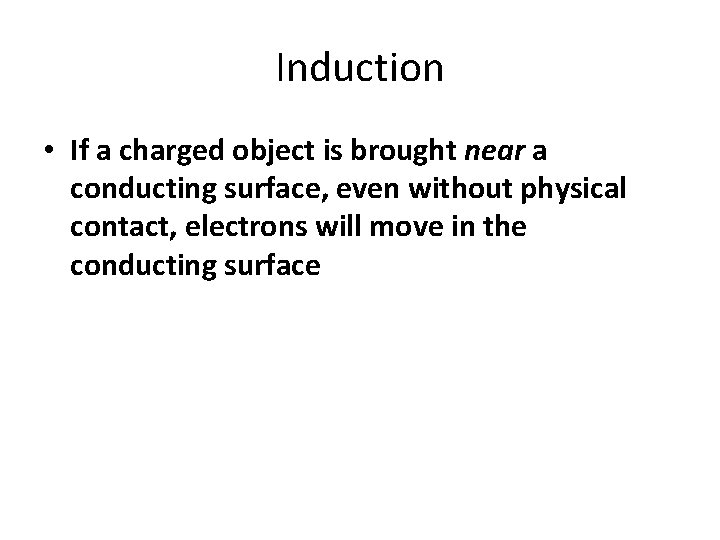Induction • If a charged object is brought near a conducting surface, even without physical contact, electrons will move in the conducting surfaceInduction • Two metal spheres are touching each other – Remember, metal is a conductor, so electrons are free to moveInduction • When a negatively charged rod is held near one sphere, electrons in the metal are repelled by the rod. • Excess negative charge has moved to the other sphere, leaving the first sphere with an excess positive charge. • The charge on the spheres has been redistributed, or induced.Induction • When the spheres are separated and the rod removed, the spheres are charged equally and oppositely. • They have been charged by induction, which is the charging of an object without direct contact • This can only work with a conductive material • No freedom of electron movement with an insulator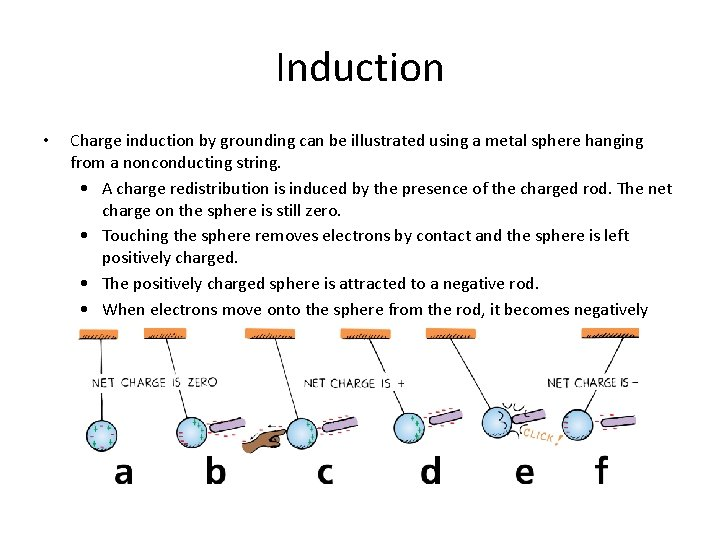Induction • Charge induction by grounding can be illustrated using a metal sphere hanging from a nonconducting string. • A charge redistribution is induced by the presence of the charged rod. The net charge on the sphere is still zero. • Touching the sphere removes electrons by contact and the sphere is left positively charged. • The positively charged sphere is attracted to a negative rod. • When electrons move onto the sphere from the rod, it becomes negatively charged by contact.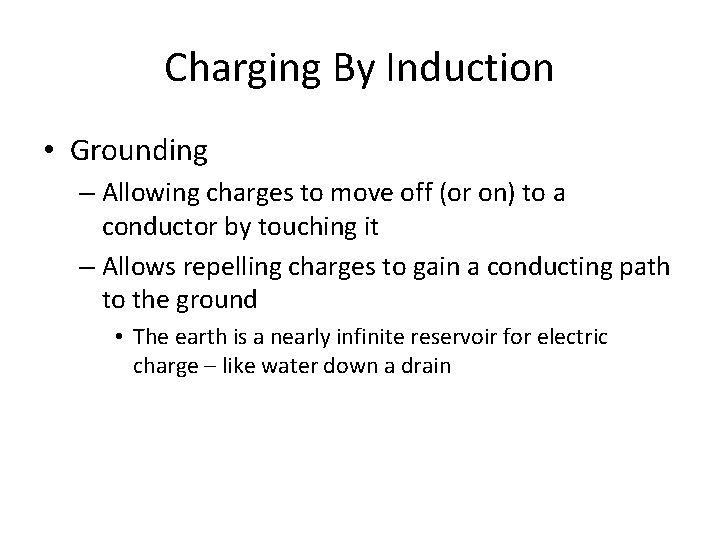Charging By Induction • Grounding – Allowing charges to move off (or on) to a conductor by touching it – Allows repelling charges to gain a conducting path to the ground • The earth is a nearly infinite reservoir for electric charge – like water down a drain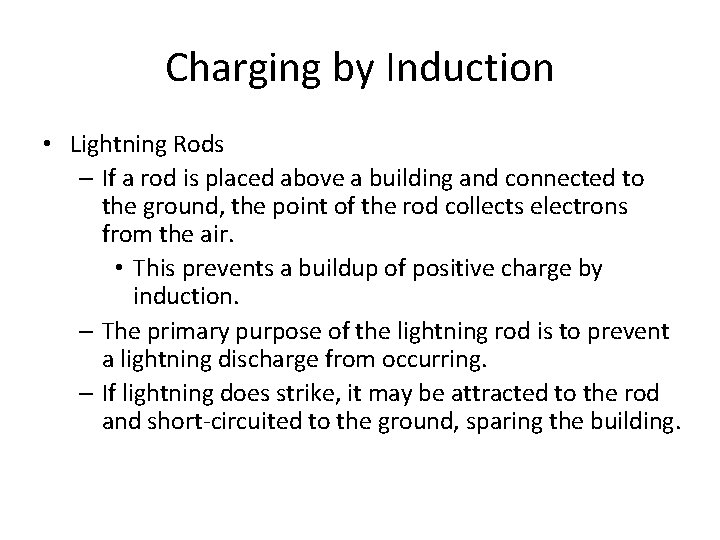Charging by Induction • Lightning Rods – If a rod is placed above a building and connected to the ground, the point of the rod collects electrons from the air. • This prevents a buildup of positive charge by induction. – The primary purpose of the lightning rod is to prevent a lightning discharge from occurring. – If lightning does strike, it may be attracted to the rod and short-circuited to the ground, sparing the building.Polarization • Charge polarization can occur in insulators that are near a charged object. • When a charged rod is brought near an insulator, there are no free electrons to migrate throughout the insulating material. – Instead, there is a rearrangement of the positions of charges within the atoms and molecules themselves.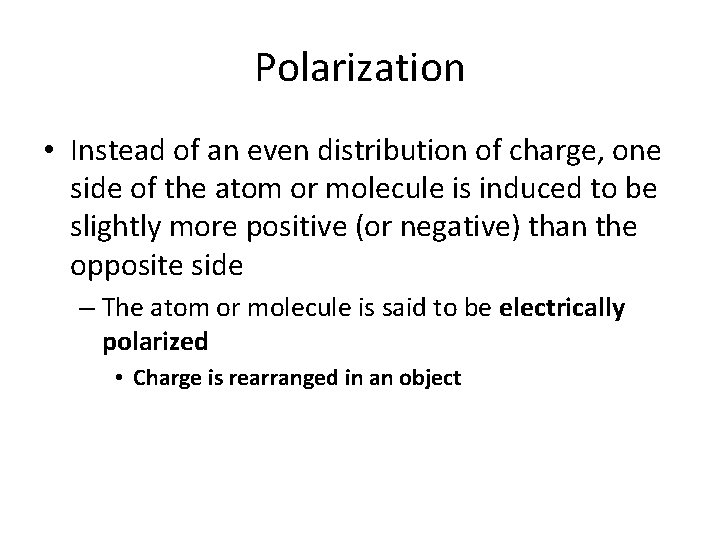Polarization • Instead of an even distribution of charge, one side of the atom or molecule is induced to be slightly more positive (or negative) than the opposite side – The atom or molecule is said to be electrically polarized • Charge is rearranged in an object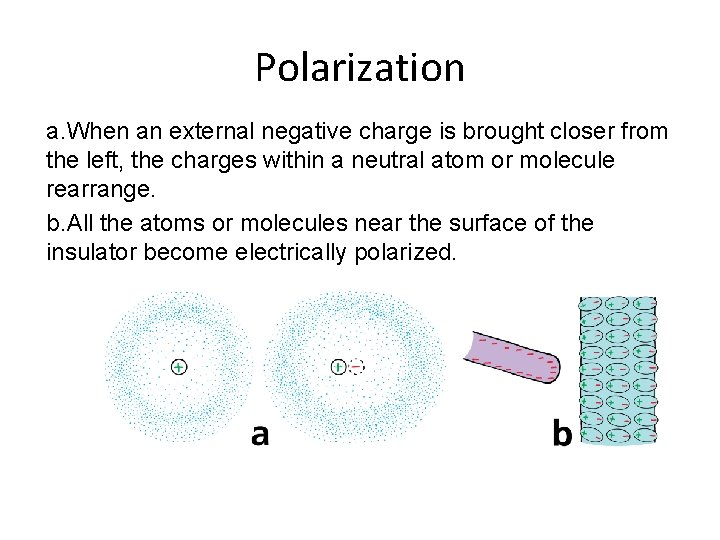Polarization a. When an external negative charge is brought closer from the left, the charges within a neutral atom or molecule rearrange. b. All the atoms or molecules near the surface of the insulator become electrically polarized.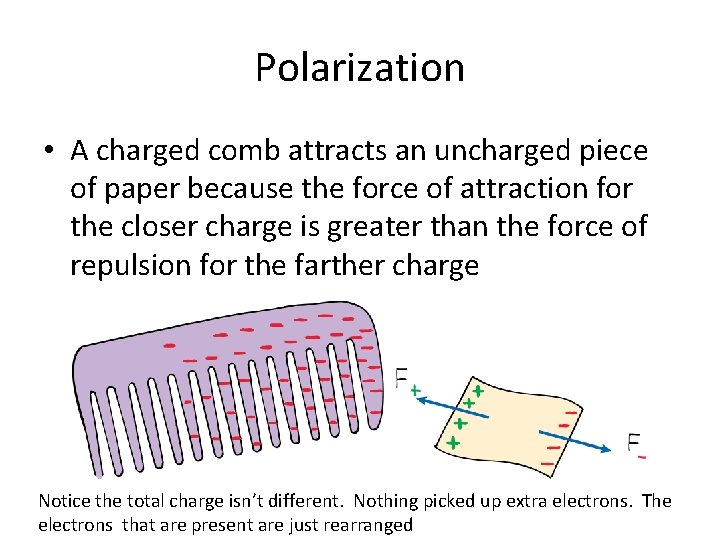Polarization • A charged comb attracts an uncharged piece of paper because the force of attraction for the closer charge is greater than the force of repulsion for the farther charge Notice the total charge isn’t different. Nothing picked up extra electrons. The electrons that are present are just rearranged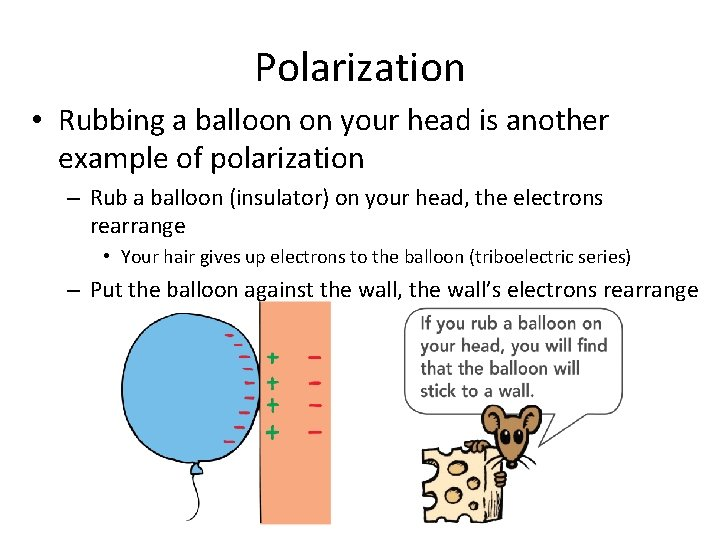Polarization • Rubbing a balloon on your head is another example of polarization – Rub a balloon (insulator) on your head, the electrons rearrange • Your hair gives up electrons to the balloon (triboelectric series) – Put the balloon against the wall, the wall’s electrons rearrangeMolecular Polarization • In chemistry, we talk about polar molecules – This occurs in covalent bonds when electrons are shared unequally in the bond – The more electronegative atom in the bond gets the electrons more of the time – This creates a partial charge in the molecule which is known as a dipole A space filling model for water is shown above. The oxygen is the more electronegative atomSummary • Objects are electrically charged – by friction • electrons transferred from one object to another – by contact • electrons are transferred from one object to another without rubbing – by induction • electrons are transferred by presence of nearby charge • NO physical contact!! • If the object is an insulator – The charge is rearranged instead of moved • Charge polarization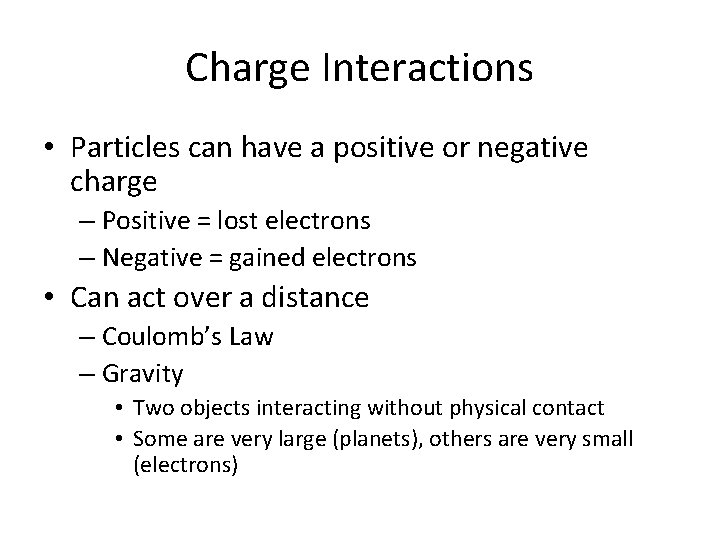Charge Interactions • Particles can have a positive or negative charge – Positive = lost electrons – Negative = gained electrons • Can act over a distance – Coulomb’s Law – Gravity • Two objects interacting without physical contact • Some are very large (planets), others are very small (electrons)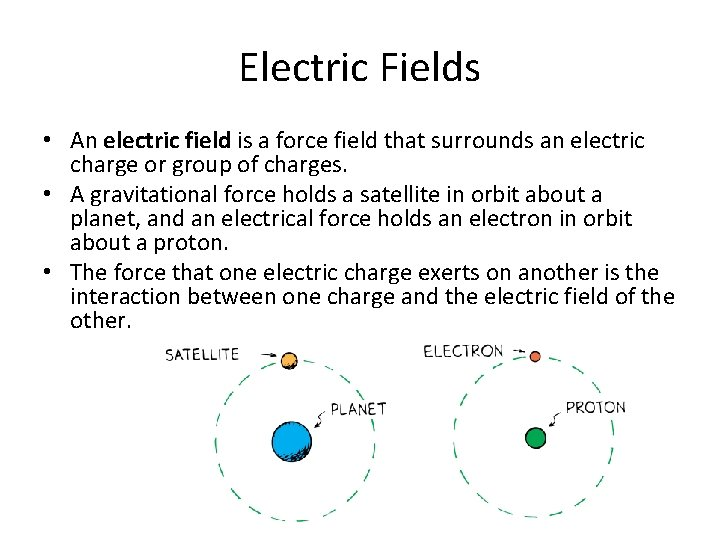Electric Fields • An electric field is a force field that surrounds an electric charge or group of charges. • A gravitational force holds a satellite in orbit about a planet, and an electrical force holds an electron in orbit about a proton. • The force that one electric charge exerts on another is the interaction between one charge and the electric field of the other.Electric Fields • Electric fields alter the space surrounding a charged object – Influences other objects in the field – Discussed earlier – Like charges repel, opposites attract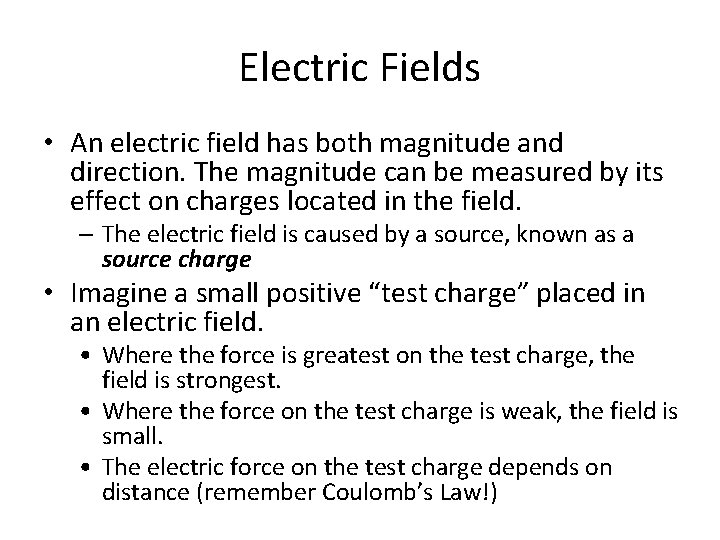Electric Fields • An electric field has both magnitude and direction. The magnitude can be measured by its effect on charges located in the field. – The electric field is caused by a source, known as a source charge • Imagine a small positive “test charge” placed in an electric field. • Where the force is greatest on the test charge, the field is strongest. • Where the force on the test charge is weak, the field is small. • The electric force on the test charge depends on distance (remember Coulomb’s Law!)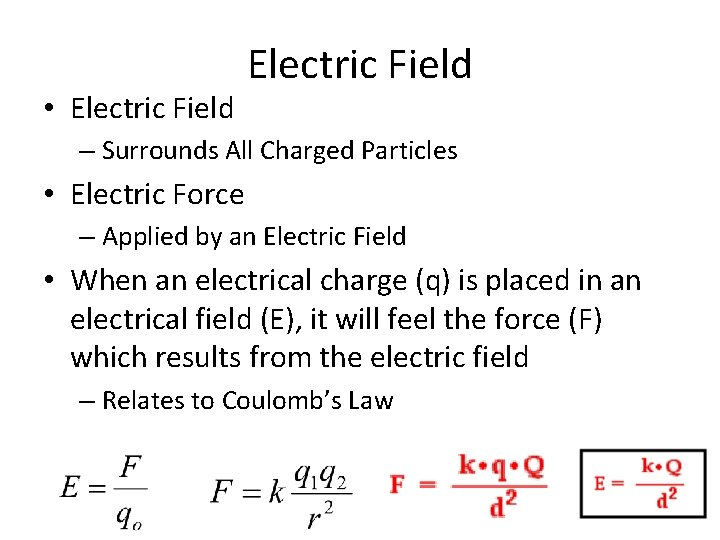• Electric Field – Surrounds All Charged Particles • Electric Force – Applied by an Electric Field • When an electrical charge (q) is placed in an electrical field (E), it will feel the force (F) which results from the electric field – Relates to Coulomb’s Law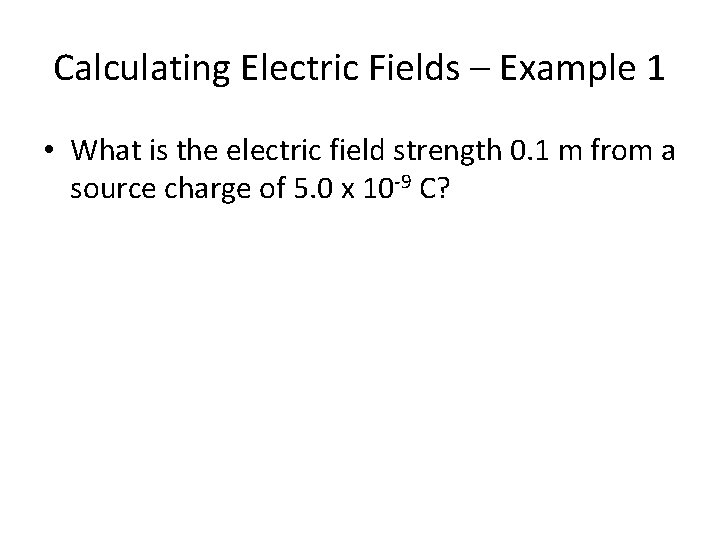Calculating Electric Fields – Example 1 • What is the electric field strength 0. 1 m from a source charge of 5. 0 x 10 -9 C?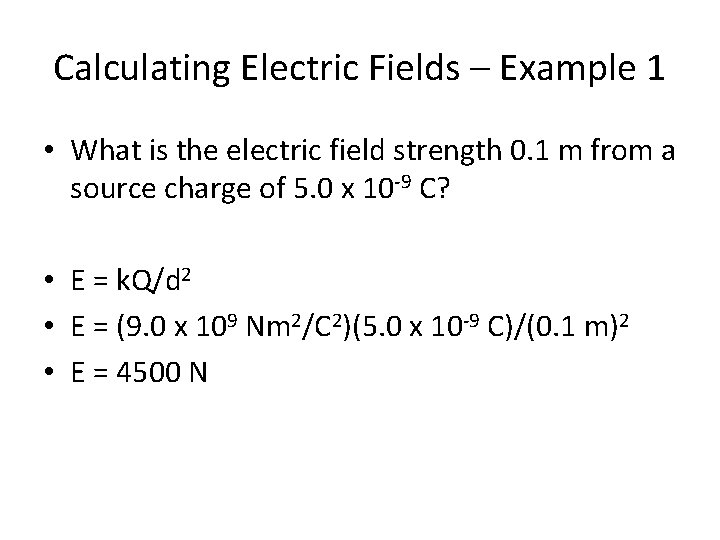Calculating Electric Fields – Example 1 • What is the electric field strength 0. 1 m from a source charge of 5. 0 x 10 -9 C? • E = k. Q/d 2 • E = (9. 0 x 109 Nm 2/C 2)(5. 0 x 10 -9 C)/(0. 1 m)2 • E = 4500 NCalculating Electric Fields – Example 2 • The electric field strength of a 1. 0 x 10 -10 C charge is 20 N. At what distance is the charge from this field strength measurement?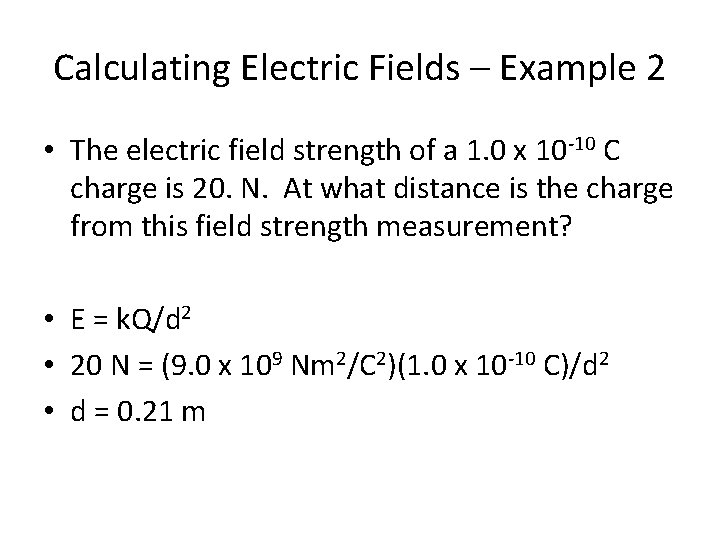Calculating Electric Fields – Example 2 • The electric field strength of a 1. 0 x 10 -10 C charge is 20. N. At what distance is the charge from this field strength measurement? • E = k. Q/d 2 • 20 N = (9. 0 x 109 Nm 2/C 2)(1. 0 x 10 -10 C)/d 2 • d = 0. 21 m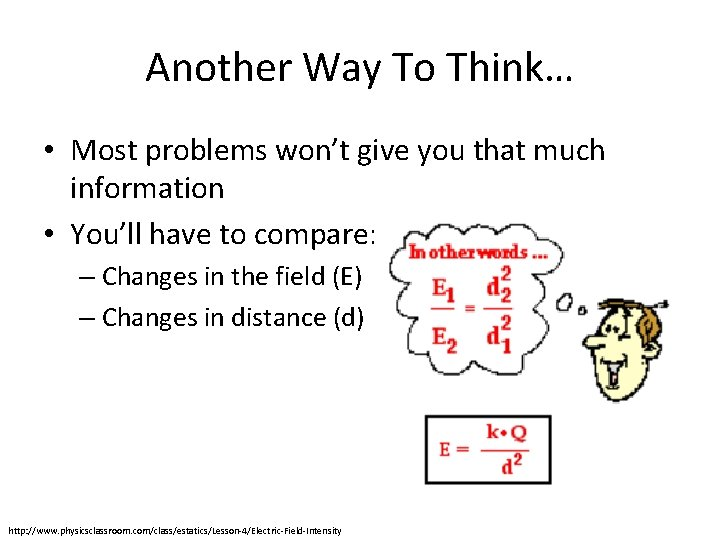Another Way To Think… • Most problems won’t give you that much information • You’ll have to compare: – Changes in the field (E) – Changes in distance (d) http: //www. physicsclassroom. com/class/estatics/Lesson-4/Electric-Field-Intensity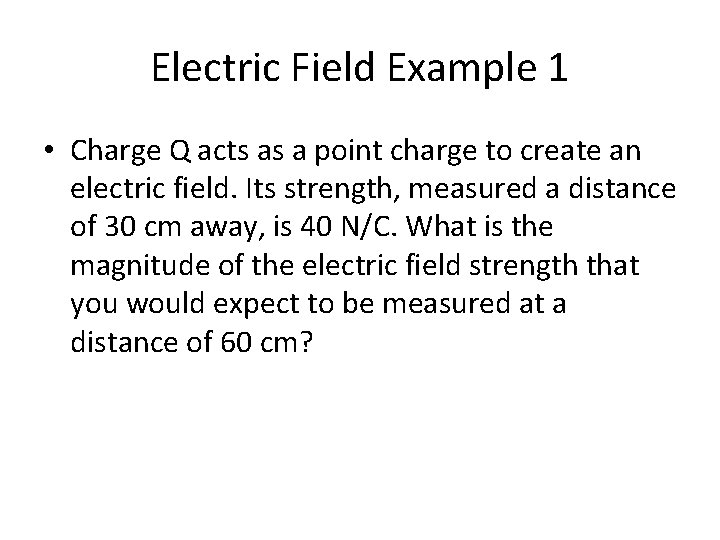Electric Field Example 1 • Charge Q acts as a point charge to create an electric field. Its strength, measured a distance of 30 cm away, is 40 N/C. What is the magnitude of the electric field strength that you would expect to be measured at a distance of 60 cm?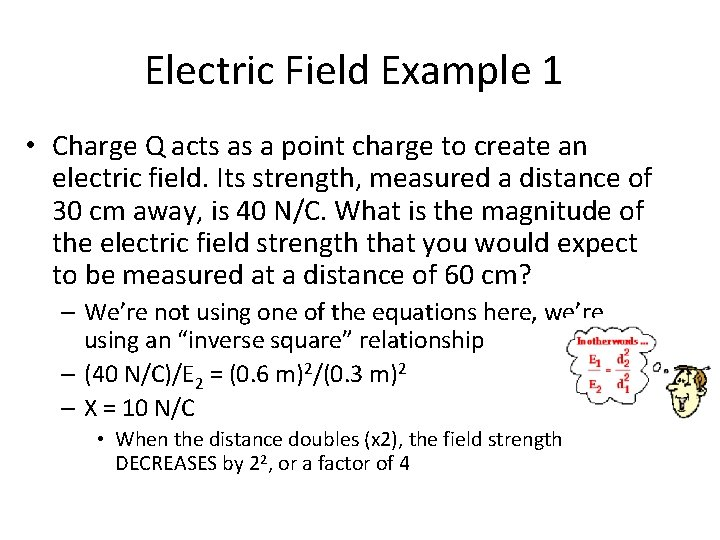Electric Field Example 1 • Charge Q acts as a point charge to create an electric field. Its strength, measured a distance of 30 cm away, is 40 N/C. What is the magnitude of the electric field strength that you would expect to be measured at a distance of 60 cm? – We’re not using one of the equations here, we’re using an “inverse square” relationship – (40 N/C)/E 2 = (0. 6 m)2/(0. 3 m)2 – X = 10 N/C • When the distance doubles (x 2), the field strength DECREASES by 22, or a factor of 4Electric Field Example 2 • Charge Q acts as a point charge to create an electric field. Its strength, measured a distance of 30 cm away, is 40 N/C. What is the magnitude of the electric field strength that you would expect to be measured at a distance of 90 cm away?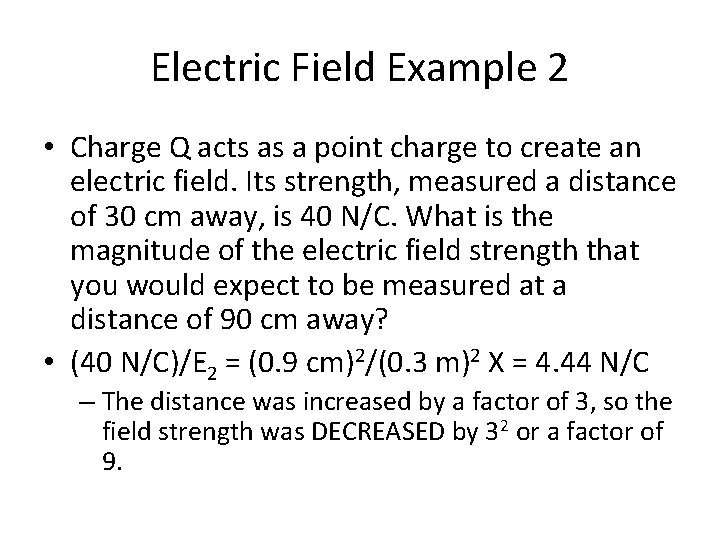Electric Field Example 2 • Charge Q acts as a point charge to create an electric field. Its strength, measured a distance of 30 cm away, is 40 N/C. What is the magnitude of the electric field strength that you would expect to be measured at a distance of 90 cm away? • (40 N/C)/E 2 = (0. 9 cm)2/(0. 3 m)2 X = 4. 44 N/C – The distance was increased by a factor of 3, so the field strength was DECREASED by 32 or a factor of 9.Electric Field Example 3 • Charge Q acts as a point charge to create an electric field. Its strength, measured a distance of 30 cm away, is 40 N/C. What would be the electric field strength. . . • a. 30 cm away from a source with charge 2 Q? • b. 60 cm away from a source with charge 2 Q? • c. 15 cm away from a source with charge 2 Q? • d. 150 cm away from a source with charge 0. 5 Q?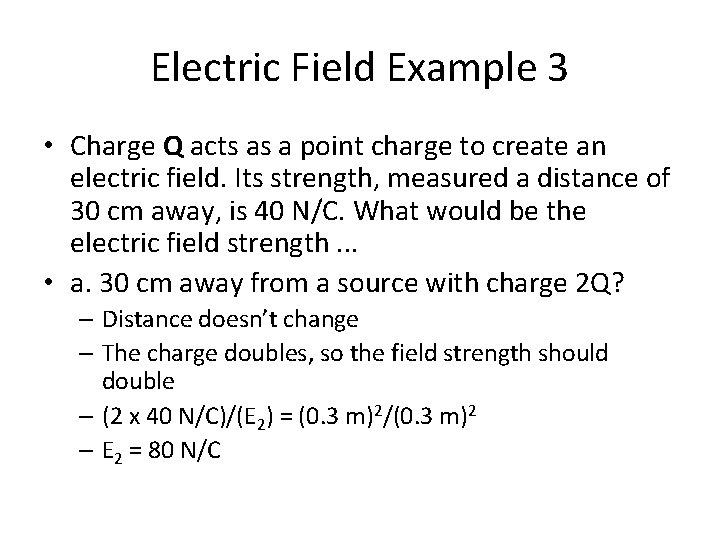Electric Field Example 3 • Charge Q acts as a point charge to create an electric field. Its strength, measured a distance of 30 cm away, is 40 N/C. What would be the electric field strength. . . • a. 30 cm away from a source with charge 2 Q? – Distance doesn’t change – The charge doubles, so the field strength should double – (2 x 40 N/C)/(E 2) = (0. 3 m)2/(0. 3 m)2 – E 2 = 80 N/C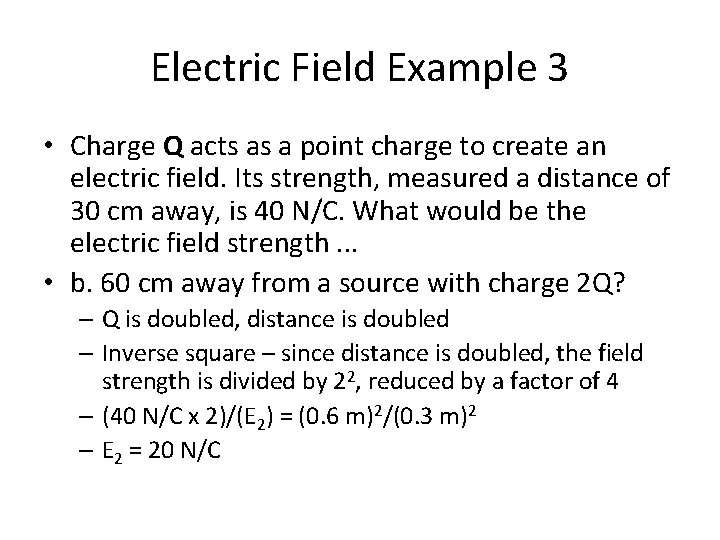Electric Field Example 3 • Charge Q acts as a point charge to create an electric field. Its strength, measured a distance of 30 cm away, is 40 N/C. What would be the electric field strength. . . • b. 60 cm away from a source with charge 2 Q? – Q is doubled, distance is doubled – Inverse square – since distance is doubled, the field strength is divided by 22, reduced by a factor of 4 – (40 N/C x 2)/(E 2) = (0. 6 m)2/(0. 3 m)2 – E 2 = 20 N/C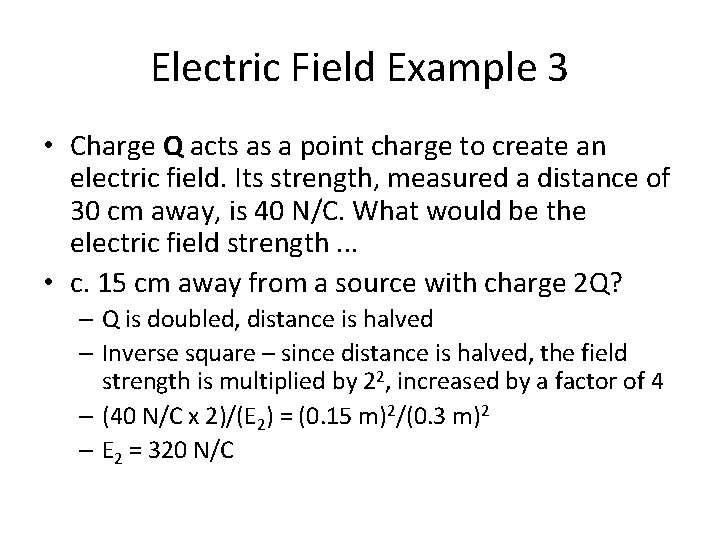Electric Field Example 3 • Charge Q acts as a point charge to create an electric field. Its strength, measured a distance of 30 cm away, is 40 N/C. What would be the electric field strength. . . • c. 15 cm away from a source with charge 2 Q? – Q is doubled, distance is halved – Inverse square – since distance is halved, the field strength is multiplied by 22, increased by a factor of 4 – (40 N/C x 2)/(E 2) = (0. 15 m)2/(0. 3 m)2 – E 2 = 320 N/CElectric Field Example 3 • Charge Q acts as a point charge to create an electric field. Its strength, measured a distance of 30 cm away, is 40 N/C. What would be the electric field strength. . . • d. 150 cm away from a source with charge 0. 5 Q? – Source charge is halved, should decrease by factor of 2 – Distance is increased by a factor of 5, strength decreases by 52 = 25 times – Combined effect is reduced by a factor of 50 (2 for source x 25 for distance) – (40 N/C x 0. 5)/(E 2) = (1. 5 m)2/(0. 3 m)2 – E 2 = 0. 8 N/C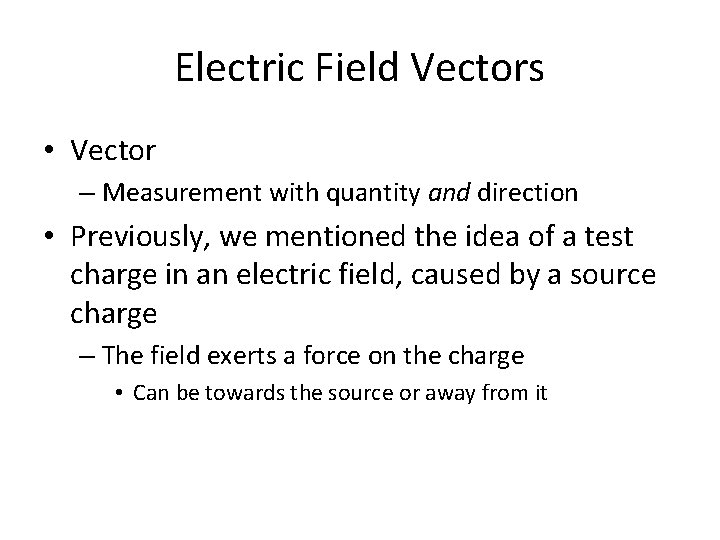Electric Field Vectors • Vector – Measurement with quantity and direction • Previously, we mentioned the idea of a test charge in an electric field, caused by a source charge – The field exerts a force on the charge • Can be towards the source or away from it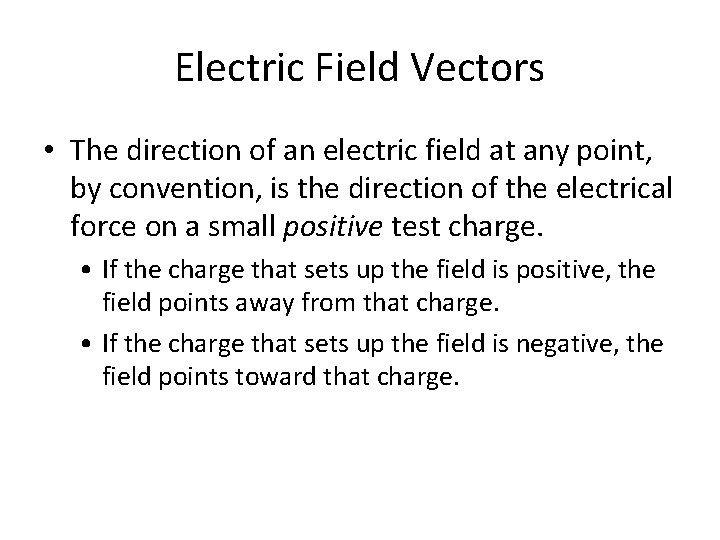Electric Field Vectors • The direction of an electric field at any point, by convention, is the direction of the electrical force on a small positive test charge. • If the charge that sets up the field is positive, the field points away from that charge. • If the charge that sets up the field is negative, the field points toward that charge.Electric Field Lines • Since an electric field has both magnitude and direction, it is a vector quantity and can be represented by vectors. • A negatively charged particle is surrounded by vectors that point toward the particle. • For a positively charged particle, the vectors point away. • The magnitude (how strong it is) of the field is indicated by the vector length. • The electric field is greater where the vectors are longer.Drawing Electric Field Lines • More lines = greater amount of charge present on test charge – Charge on A < charge on B < charge on C – Is this charge + or - ? http: //www. physicsclassroom. com/class/estatics/Lesson-4/Electric-Field-Lines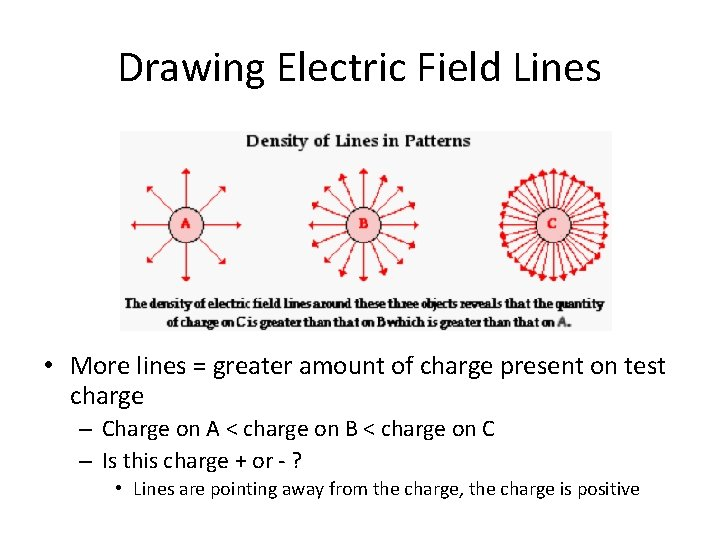Drawing Electric Field Lines • More lines = greater amount of charge present on test charge – Charge on A < charge on B < charge on C – Is this charge + or - ? • Lines are pointing away from the charge, the charge is positive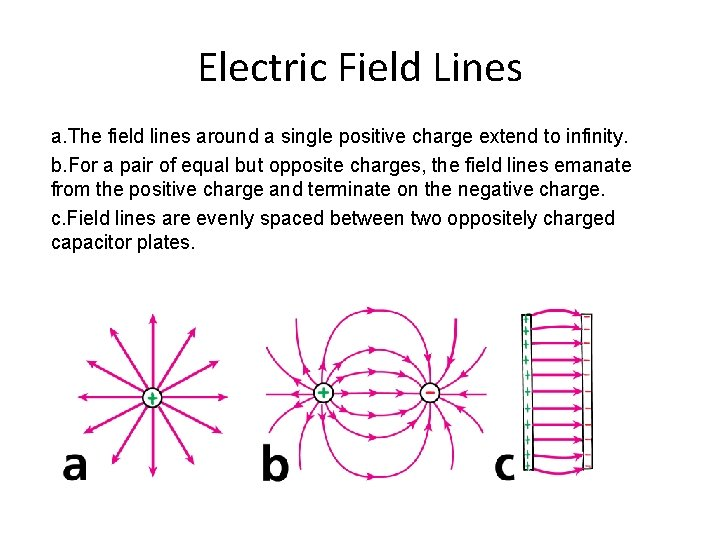Electric Field Lines a. The field lines around a single positive charge extend to infinity. b. For a pair of equal but opposite charges, the field lines emanate from the positive charge and terminate on the negative charge. c. Field lines are evenly spaced between two oppositely charged capacitor plates.Electric Field Lines • Lines are ALWAYS perpendicular to the source, never parallel – Would cause an acceleration of charge and cause current • Lines never cross https: //willthethrillonthebeach. files. wordpress. com/2014/09/img_0588. gifElectrostatic Equilibrium • One characteristic of a conductor at electrostatic equilibrium is that the electric field anywhere beneath the surface of a charged conductor is zero. – Electric field lines are only on the outer surface. – The lines extend from this surface outward, not inward. – Assumes no electric charge inside the conductor http: //www. physicsclassroom. com/class/estatics/Lesson-4/Electric-Fields-and-ConductorsFaraday’s Cage • Michael Faraday (1791 -1867) – Discovered the concept of using metals to shield from electric fields – Constructed a room within an electric field shielded by metal foil • Known as “Faraday’s cage” https: //backyardbrains. com/experiments/img/Fig 2_Sketch_Faraday. Cage_Fields. jpeg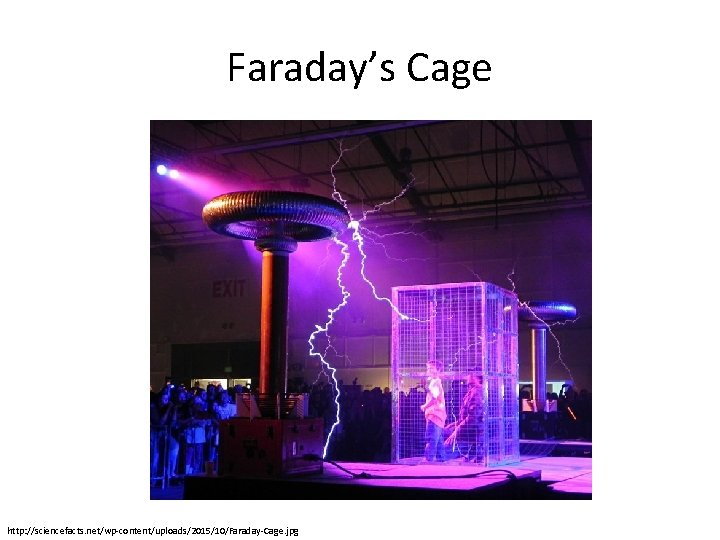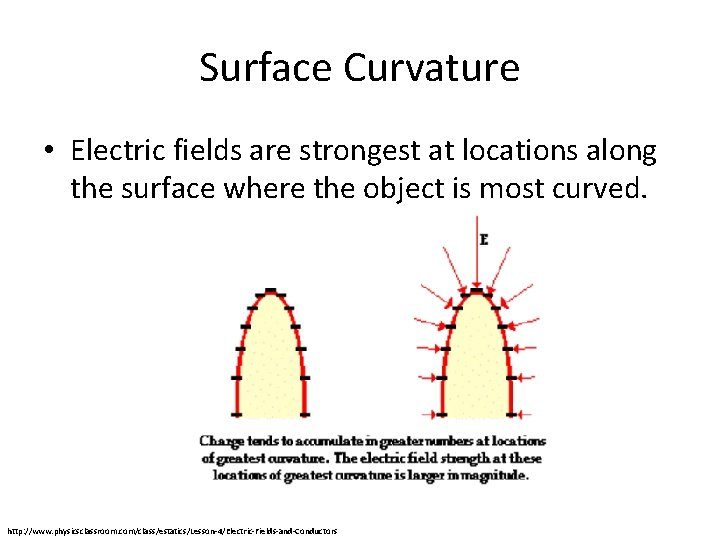Surface Curvature • Electric fields are strongest at locations along the surface where the object is most curved. http: //www. physicsclassroom. com/class/estatics/Lesson-4/Electric-Fields-and-ConductorsLightning • The precursor of any lightning strike is the polarization of positive and negative charges within a storm cloud – Frictional charging from the evaporation and collision of water vapor with the cloud • Electrons come off during this processLightning • The precursor of any lightning strike is the polarization of positive and negative charges within a storm cloud – Freezing process • Cold clouds cause water vapor to freeze • Ice crystals in the cloud sink • Frozen portion is negatively charged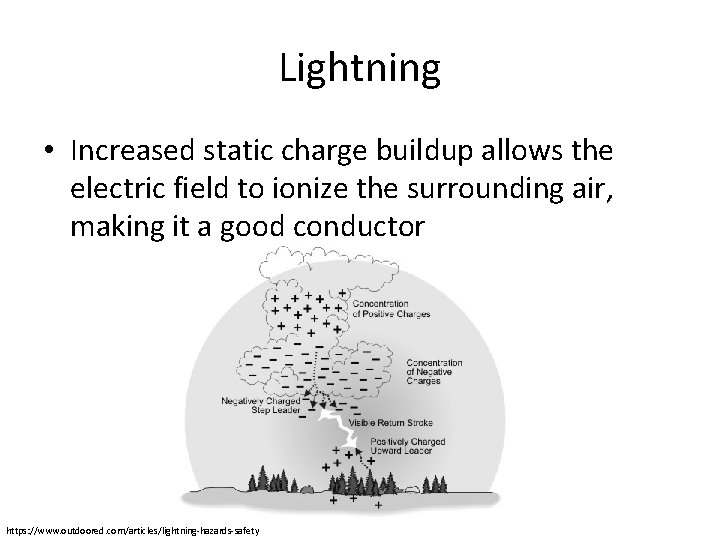Lightning • Increased static charge buildup allows the electric field to ionize the surrounding air, making it a good conductor https: //www. outdoored. com/articles/lightning-hazards-safety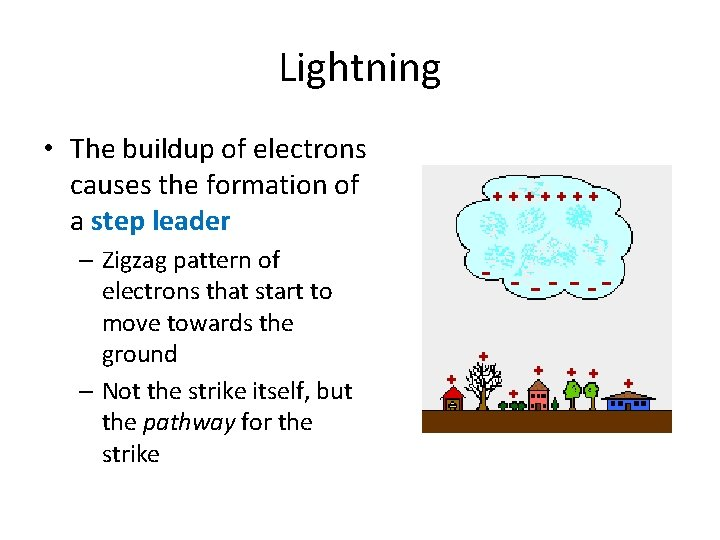Lightning • The buildup of electrons causes the formation of a step leader – Zigzag pattern of electrons that start to move towards the ground – Not the strike itself, but the pathway for the strike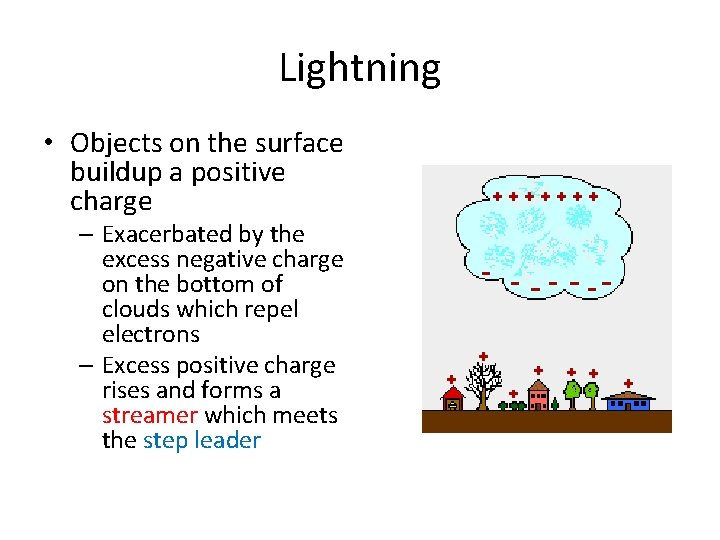Lightning • Objects on the surface buildup a positive charge – Exacerbated by the excess negative charge on the bottom of clouds which repel electrons – Excess positive charge rises and forms a streamer which meets the step leaderLightning • When step leader (-) meets streamer (+), the pathway is completed and the lightning strike occurs – A strike may contain up to 1021 electrons travelling in less than a millisecond